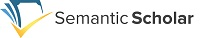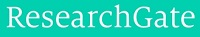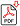Search for Articles:

 Indexing RepositoriesSponsors## Topological Venn Diagrams and Graphs

T. Jean Brice, G. Easwara Prasad, G. Selvam

Abstract: This paper is an attempt to expound Venn diagrams and its applications in Topology and Graph Theory. John Venn introduced Venn diagrams in 1880. Venn diagrams can be called as Eulerian Circles. Euler invented this in the 18th century. Venn diagram consists of curves and circles. Venn diagrams are similar to Euler diagrams. Edwards discusses the dual graph of a Venn diagram is a maximal planar subgraph. Edwards-Venn diagrams are topologically equivalent to diagrams with graphs. 2D and 3D Venn diagrams consist of labeled simple closed curves. 3D Venn diagrams and 3D Euler diagrams are all combinations of surface intersections. In brief this paper will give a vivid picture of Venn diagrams and its close relationship with Topology and Graph Theory.

Keywords: Venn diagram ? Euler diagram ? planar Venn diagram ? Topologically faithful Venn diagram ? 2D and 3D Venn diagram.

Country: India, Subject Area: Mathematics

Pages: 1436 - 1440

Edition: Volume 8 Issue 5, May 2019